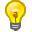# JavaScript Number 对象

2022-05-18 15:05 更新

JavaScript 的 Number 对象是经过封装的能让你处理数字值的对象，由 Number() 构造器创建。

JavaScript 只有一种数字类型。

## JavaScript 数字

JavaScript 数字可以使用也可以不使用小数点来书写：

## 实例

var pi=3.14;    // 使用小数点
var x=34;       // 不使用小数点

## 实例

var y=123e5;    // 12300000
var z=123e-5;   // 0.00123

## 所有 JavaScript 数字均为 64 位

JavaScript 不是类型语言。与许多其他编程语言不同，JavaScript 不定义不同类型的数字，比如整数、短、长、浮点等等。

52 bits (0 - 51)  11 bits (52 - 62) 1 bit (63)

## 实例

var x = 0.2+0.1; // result will be 0.30000000000000004

## 实例

var y = 0377;
var z = 0xFF;绝不要在数字前面写零，除非您需要进行八进制转换。

## 实例

var myNumber=128;
myNumber.toString(16);   // returns 80
myNumber.toString(8);    // returns 200
myNumber.toString(2);    // returns 10000000

## 实例

myNumber=2;
while (myNumber!=Infinity)
{
myNumber=myNumber*myNumber; // Calculate until Infinity
}

var x = 2/0;
var y = -2/0;

## NaN - 非数字值

NaN 属性是代表非数字值的特殊值。该属性用于指示某个值不是数字。可以把 Number 对象设置为该值，来指示其不是数字值。

## 实例

var x = 1000 / "Apple";
isNaN(x); // returns true
var y = 100 / "1000";
isNaN(y); // returns false

## 实例

var x = 1000 / 0;
isNaN(x); // returns false

## 数字可以是数字或者对象

JavaScript 数字对象初始化数据， var y = new Number(123);

## 实例

var x = 123;
var y = new Number(123);
typeof(x) // returns Number
typeof(y) // returns Object

## 实例

var x = 123;
var y = new Number(123);
(x === y) // is false because x is a number and y is an object.

## 数字属性

• MAX_VALUE
• MIN_VALUE
• NEGATIVE_INFINITY
• POSITIVE_INFINITY
• NaN
• prototype
• constructor

## 数字方法

• toExponential()
• toFixed()
• toPrecision()
• toString()
• valueOf()

## 相关文章

JavaScript 和 HTML DOM 参考手册：JavaScript Number 对象

App下载